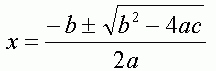SEARCH HOMEMath Central Quandaries & QueriesQuestion from Ash, a student: Y = -1/100X^2 =27/10X +0 = 0 THE Y INTERCEPT IS 50 HOW DO I FIND THE X INTERCEPTTo find the x-intercept, also known as the "zero", or "root" of the equation, set the value of y to 0, and solve the resulting quadratic equation using the quadratic formula.  For an equation of the form

y = a(x2) + bx + c           Where a, b and c are constant numbers (as opposed to variables),

the quadratic equation iswhere ± means (+) OR (-).  Therefore there are two roots to a quadratic equation, one given by using the (+) and one given by using the (-).  Therefore there "usually" are 2 y-intercept values of a graph involving x2.

I say "usually", because you will notice that in the quadratic formula, there is a square root.  In general, nothing prevents the value of 4ac from being greater than b2, in which case you would be taking the square root of a negative number, which is not a real number.  If this is the case, there are NO y-intercepts.  Also, you could have the situation where b2 = 4ac, in which case you would be adding or subtracting 0 in the quadratic equation.  This would mean that there is only one y-intercept.

Hope this is helpful!
GabeMath Central is supported by the University of Regina and The Pacific Institute for the Mathematical Sciences.